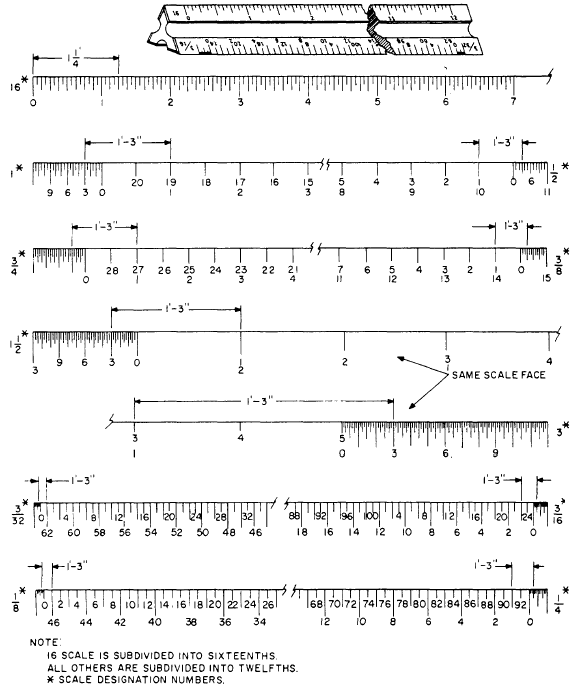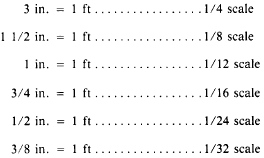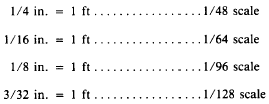Custom SearchBeam CompassBasic EngineeringEngineers ScaleArchitects Scale

ARCHITECTS SCALES are usually tri-angular in shape and are used wherever dimensions are measured in feet and inches. Major divisions on the scale represent feet which, in turn, are subdivided into 12ths or 16ths, depending on the individual scale.Figure 2-24.-Architects scale.

Figure 2-24 shows the triangular architects scale. Also shown are segments of each of the eleven scales found on this particular type of scale. Notice that all scales except the 16th scale are actually two scales that read from either left to right or right to left. When reading a scale numbered from left to right, notice that the numerals are located closer to the outside edge. On scales that are numbered from right to left, notice that the numerals are located closer to the inside edge.

Architects scales are "open" divided (only the main divisions are marked throughout the length) with the only subdivided interval being an extra interval below the 0-ft mark. These extra intervals are divided into 12ths. To make a scale measurement in feet and inches, lay off the number of feet on the main scale and add the inches on the subdivided extra interval. However, notice that the 16th scale is fully divided with its divisions being divided into 16ths.

Now lets measure off a distance of 1 ft 3 in. to see how each scale is read and how the scales compare to one another. (Refer to fig. 2-24.) Since the graduations on the 16th scale are subdivided into 16ths, we will have to figure out that 3 in. actually is 3/12 or 1/4 of a foot. Changing this to 16ths, we now see we must measure off 4/16ths to equal the 3-in. measurement. Note carefully the value of the graduations on the extra interval, which varies with different scales. On the 3 in. = 1 ft scale, for example, the space between adjacent graduations represents one-eighth in. On the 3/32 in. = 1 ft scale, however, each space between adjacent graduations represents 2 in. The scale 3/32 in. = 1 ft, expressed fraction-ally, comes to 3/32 = 12, or 1/128. This is the smallest scale provided on an architects scale. The scales on the architects scale, with their fractional equivalents, are as follows:Integrated Publishing, Inc. - A (SDVOSB) Service Disabled Veteran Owned Small Business